# Rational Numbers Class 8 Notes

In this Rational Numbers Class 8 Notes Page we will explain the topics for the chapter 1 of Rational numbers Class 8 Maths.We have given quality notes and video to explain various things so that students can benefits from it and learn maths in a fun and easy manner, Hope you like them and do not forget to like , social share and comment at the end of the page.
Table Of content

## Introduction to Rational Numbers

Counting Numbers/Natural Numbers
The numbers 1,2,3,… are called natural numbers or counting numbers.

Whole Numbers
Let us add one more number i.e. ,zero(0), to the collection of natural numbers.
Now the numbers are 0,1,2,… These numbers are called whole numbers

We can say that whole numbers. consist of zero and the natural numbers. Therefore, except zero all the whole numbers. are natural numbers.
So all natural Number are whole number but all whole numbers are not natural numbers
Integers
Z={…-7,-6,-5,-4,-3,-2,-1,0,1,2,3,4,5,6…..}

Rational Numbers Example :  ½ , 4/3 ,5/7 ,1 etc.

## Properties of Rational Numbers

### Closure Property

The Closure Property states that when you perform an operation (such as addition, multiplication, subtraction etc) on any two numbers in a certain type of number, the result of the computation is another number in the same type of number
Here is the summary for the closure property on various mathematical operations on various numbers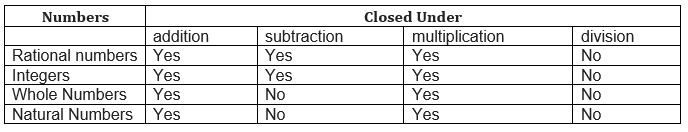Watch this tutorial for more explanation about closure property

### Commutativity Property

The Commutativity Property states that order does not matter so if you swap numbers in an operation (such as addition, multiplication, subtraction etc) on any two numbers in a certain type of number, the result of the computation is same as the result without swap
Here is the summary for the commutative property on various mathematical operations on various numbers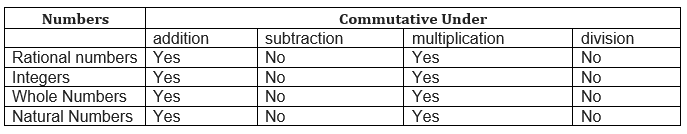Watch this tutorial for more explanation about commutative property

### Associativity Property

The Associativity Property states that it doesn't matter how we group the numbers (i.e. which we calculate first) .We can add parenthesis any where and we get the same answer
Here is the summary for the Associative property on various mathematical operations on various numbers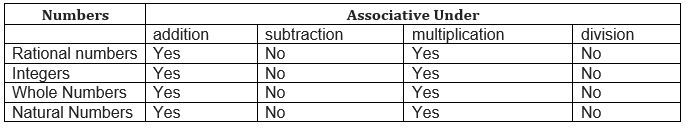Watch this tutorial for more explanation about Associative property

We observe
a + 0 = 0 + a = a, where a is a whole number
b + 0 = 0 + b = b, where b is an integer
c + 0 = 0 + c = c, where c is a rational number
Zero is called the identity for the addition of rational numbers. It is the additive identity for integers and whole numbers as well

## Multiplicative identity/Role of one

We observe
a × 1 =  = a, where a is a whole number
b × 1 = b, where b is an integer
c × 1 = c, where c is a rational number
1 is the multiplicative identity for rational numbers. It is the multiplicative identity for integers and whole numbers as well

We observe
a + (-a) = 0, where a is a whole number
b +(-b)  = 0, where b is an integer
(a/b) + (-a/b) = 0, where a/b is a rational number
So we say that (-a/b) is the additive inverse of a/b   and a/b is the additive inverse for (-a/b)

Example
What is the additive inverse of following numbers
a) 1/6
b) -8/9
Solution:
Additive Inverse of any rational number a/b is defined as -a/b  so that (a/b) +(-a/b)=0
So   for 1/6, additive inverse will be -1/6
Similarly for -8/9 ,it will be  8/9

## Reciprocal or multiplicative inverse

The multiplicative inverse of any rational number a/b is defined as b/a  so that (a/b) x (b/a) =1
Zero does not have any reciprocal or multiplicative inverse
Example
Find the multiplicative inverse of the following.
-54
-11/12
Solution
The multiplicative inverse of any rational number a/b is defined as b/a  so that (a/b) x (b/a) =1
-54=-54/1 multiplicative inverse will 1/-54 =-1/54
Similarly for -11/12, the multiplicative inverse will be -12/11

## Distributivity of Multiplication over Addition and Subtraction.

For all rational numbers a, b and c,
a (b + c) = ab + ac
a (b – c) = ab – ac

## How to Represent Rational Number on the Number Line

We already know from last grades on how to represent Natural numbers, whole Numbers and integers on the Number Line
Natural Numbers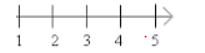Whole Numbers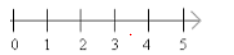Integers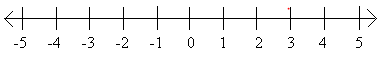Rational numbers can also be represented on the Number line
Lets take an example of 4/9
Step (1) In a rational number, the numeral below the bar, i.e., the denominator, tells the number of equal parts into which the first unit has been divided. The numeral above the bar i.e., the
numerator, tells ‘how many’ of these parts are considered
So in this particular example, we need to divide the first unit into 9 parts and we need to move to  the 4th part
Step (2) Draw the straight line.  Divide the first unit into equal parts equal to the denominator of the rational number. And then mark each equal part to the right of the line and reach the desired number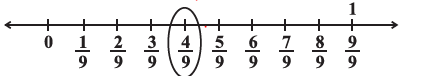## Rational Numbers between Two Rational Numbers

There are countless rational number between two rational number.
Now we will see some example to find the rational number between the rational numbers
Example 1
Find the 3 rational number between  1 and 2
Solution
Method 1  Average method
We know the average of two rational number lies between them and it is a rational number
Step 1:  First average  =(1+2)/2=3/2
So 3/2 lies between 1 and 2
Step 2:  Average of 1  and 3/2 will also lie between 1 and 3/2 and hence 1 and 2,
So =1/2 (1+3/2  )=5/4
Step 3.: Again Average of 3/2 and 2  will also lie between 1 and 2.  So 1/2 (3/2+2)=7/4
So  3/2,5/4 and 7.4 are rational Number
In General If a and b are two rational numbers, then  (a+b)/2  is a rational number between a and b such that a < (a+b)/2< b.
Method 2
We can write the number like  10/10 , 20/10
So the rational number would 11/10, 12/10, 13/10,14/10,15/10
Example 2
Find the 3 rational number between  1/3 and 7/2
Solution
Method 1  Average method
We know the average of two rational number lies between them and it is a rational number
So  step 1:  First average  =(1/3+7/2)/2=23/24
So 23/24 lies between 1/3 and 7/2
Step 2:  Average of 1/3  and 23/24 will also lie between 1/3 and 23/24 and hence 1/3 and 7/2
So =  ½(1/3+23/24)=31/48
Step 3.: Again Average of 23/24 and 7/2  will also lie between 1/3 and 7/2.  So=1/2(23/24+7/2)=107/48
Method 2:  Another simple way would be to convert the denominator into same value
1/3  =2/6
7/2=21/6
Now   2/6 <3/6 < 4/6< 5/6 …..< 20/6   < 21/6
So This way we can easy find  the rational numbers

## Frequently asked Questions on CBSE Class 8 Maths Chapter 1: Rational Numbers

### Is 0 a rational number?

Yes 0 is a rational number as it ca expressed in the form p/q i.e 0/1

### What are the types of rational numbers?

Rational numbers includes fractions,Integers, whole numbers, Natural numbers, terminating decimals numbers, Non terminating repeating decimals

### Are rational numbers finite or infinite?

Ratonal numbers are infinite.

### How to Identify a Rational Number?

Any number which can be expressed in the form p/q where p and q are integers is a Rational number. So when numbers with out decimals are given, you can easily identify using this definition Example 1/2. $\sqrt 3$ . 1/2 is Rational number as it can expressed as p/q , while $\sqrt 3$ is not rational as it cannot be expressed as p/q For decimals Numbers, If the number is terminating, then it is Rational number. If it is non terminating and repeating, then it is Rational Number. If it is non terminating and no repeating, then it is not Rational Number

### What is the identity of rational numbers?

1 is the identifty

### What are the seven properties of Rational Number?

Properties of Rational Number (i) Closure Property (ii) Commutative Property (iii) Associative Property (iv) Additive identity (v)Multiplicative Identity (vi)Additive Inverse (vii) Multipicative Inverse

## Summary

Here is Rational Numbers Class 8 Notes Summary
1. Rational number are closed under the operations of addition, subtraction and multiplication.
2. The rational number 0 is the additive identity for rational numbers while The rational number 1 is the multiplicative identity for rational number
3. The additive inverse and Multiplicative inverse of a/b is -a/b and b/a respectively
4. There are infinite Rational number between two rational numbers